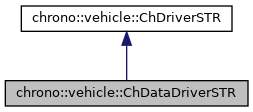## Description

Driver inputs for a suspension test rig from data file.

A driver model based on user inputs provided as time series. If provided as a text file, each line in the file must contain:

  time | steering | left_post0 right_post0 | left_post1 right_post1 | ...


It is assumed that the time values are unique. Inputs for post_left, post_right, and steering are assumed to be normalized in the range [-1,1]. Driver inputs at intermediate times are obtained through cubic spline interpolation.

#include <ChDataDriverSTR.h>

Inheritance diagram for chrono::vehicle::ChDataDriverSTR:[legend]
Collaboration diagram for chrono::vehicle::ChDataDriverSTR:[legend]

## Public Member Functions

ChDataDriverSTR (const std::string &filename)
Construct using data from the specified file.

virtual bool Ended () const override
Return true when driver stopped producing inputs (end of data).Public Member Functions inherited from chrono::vehicle::ChDriverSTR
double GetSteering () const
Get the driver steering input (in the range [-1,+1]).

const std::vector< double > & GetDisplacementLeft () const
Get the left post vertical displacement inputs (each in the range [-1,+1]).

const std::vector< double > & GetDisplacementRight () const
Get the right post vertical displacement inputs (each in the range [-1,+1]).

const std::vector< double > & GetDisplacementSpeedLeft () const
Get the left post displacement rates of change.

const std::vector< double > & GetDisplacementSpeedRight () const
Get the right post displacement rates of change.

bool Started () const
Return false while driver inputs are ignored (while the rig is reaching the ride height configuration) and true otherwise. More...

bool LogInit (const std::string &filename)
Initialize output file for recording driver inputs.

bool Log (double time)
Record the current driver inputs to the log file.

## Additional Inherited MembersProtected Member Functions inherited from chrono::vehicle::ChDriverSTR
void SetDisplacementLeft (int axle, double val, double min_val=-1, double max_val=1)
Set the value for the driver left post displacement input.

void SetDisplacementRight (int axle, double val, double min_val=-1, double max_val=1)
Set the value for the driver right post displacement input.

void SetSteering (double val, double min_val=-1, double max_val=1)
Set the value for the driver steering input.Protected Attributes inherited from chrono::vehicle::ChDriverSTR
int m_naxles
number of actuated axles

std::vector< double > m_displLeft
current value of left post displacements

std::vector< double > m_displRight
current value of right post displacements

std::vector< double > m_displSpeedLeft
current value of left post displacement rates of change

std::vector< double > m_displSpeedRight
current value of right post displacement rates of change

double m_steering
current value of steering input

double m_delay
time delay before generating inputs

The documentation for this class was generated from the following files: